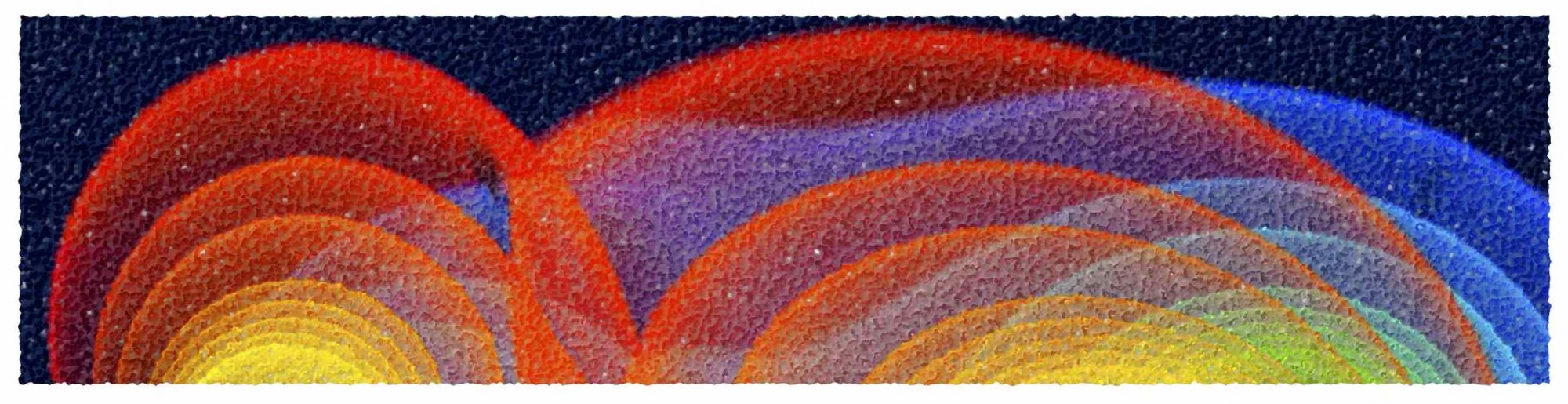# Numerical Relativity

SS2014, Tue, 14:00-16:00, Room 2.216 (main lecture); Thur, 10:00-11:00, Room 2.216 (exercises by Dr. Galeazzi)

Assigned Exercises; Scanned Lecture Notes

This is a course on advanced general relativity and provides an introduction to the mathematical and numerical techniques presently employed for the accurate solution of the Einstein equations together with those of relativistic hydrodynamics. The first part of the course will concentrate on the mathematical aspects of numerical relativity. These include the formulation of the equations, be it the field equations or those of relativistic hydrodynamics, the definition of hyperbolic system of partial differential equations and the development of nonlinear waves in hydrodynamics. The second part of the course, instead, will concentrate on the numerical aspects and the most advanced techniques for the numerical solution of these equations. The students are expected to be familiar with the theory of General Relativity and to be proficient in differ- ential geometry and tensor calculus. A series of exercises parallels the course. A detailed syllabus and a list of references can be found here.